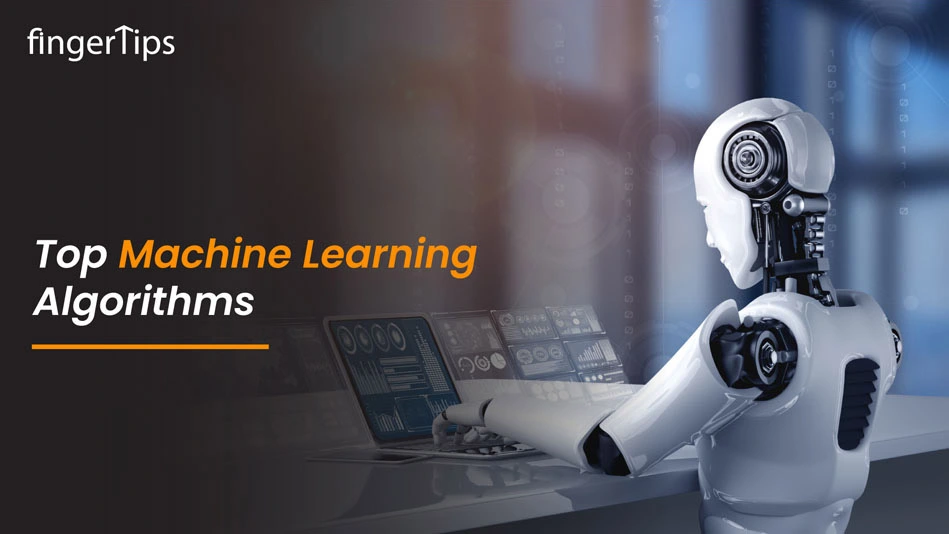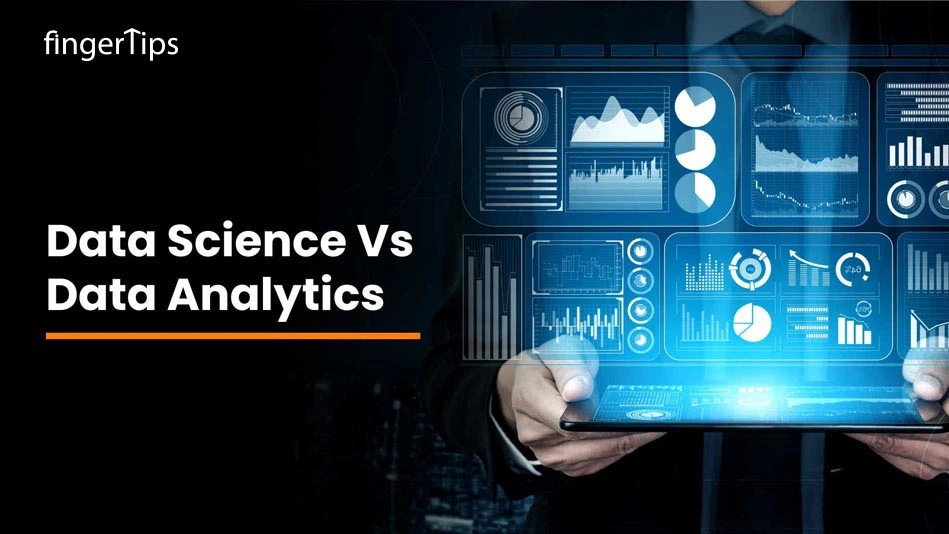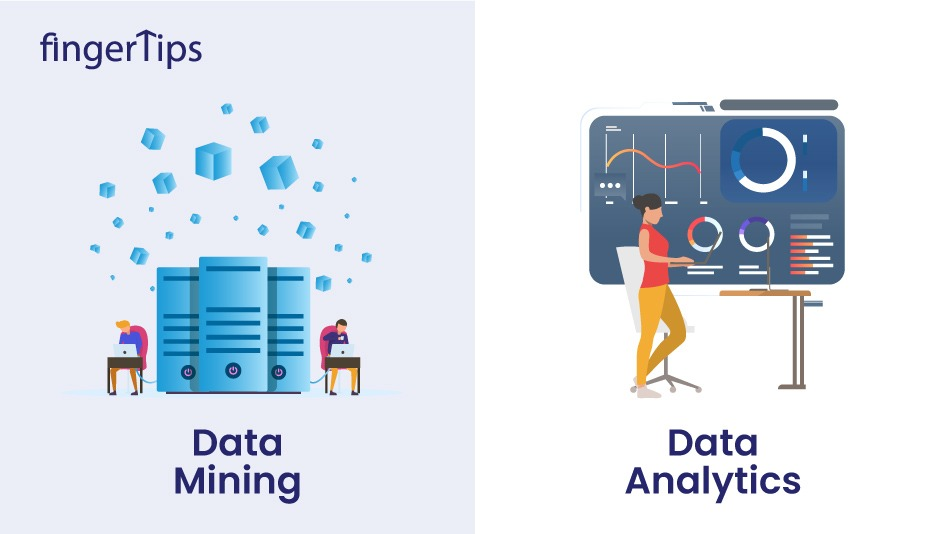Home > Blog > Data Science > Top Machine Learning Algorithms: Supervised, Unsupervised Learning, And More

# Top Machine Learning Algorithms: Supervised, Unsupervised Learning, And More

14 Nov 2022
2001### Related TopicsInterested in this course?## Introduction

Due to the enormous demand and technological improvements over the past few years, machine learning has become much more common. Machine learning has been enticing to companies across a wide range of industries due to its capacity to extract value from data. With some tweaking and modest modifications, off-the-shelf machine learning algorithms are used in the design and implementation of the majority of machine learning products.
Currently, we are in one of the most defining phases of technology and an era that is in a continuously progressing stage, computing is getting advanced over the years and we can easily predict what’s coming ahead, now we have PCs, robots, IoT devices, and self-driving cars.
The democratization of resources and methods is what excites people about this era. Thanks to Machine Learning Algorithms, data processing that used to take days can now be completed in a matter of minutes. This is the main reason for increasing the demand for Data scientists.

## What is Machine Learning?

Machine learning is a term where a machine automatically learns from different examples and experiences without being programmed.
It is a branch of artificial intelligence (AI) that mainly focuses on using data and algorithms to simulate how humans learn, gradually increasing the accuracy of the system.
Also, machine learning is an important component in the growing field of data science. Algorithms are trained using statistical techniques to produce classifications or predictions and to find important insights in data mining projects. The decisions made as a result of these insights influence key growth indicators in applications and enterprises, ideally. Data scientists will be more in demand as big data continues to develop and flourish. They will be expected to assist in determining the business questions and the information needed to address them.

The majority of the time, machine learning algorithms are developed utilizing accelerated solution development frameworks like TensorFlow and PyTorch.

## What is a Machine Learning Algorithm?

Machine learning algorithms are as same as a regular set of algorithms, what they do is program your machine in a smarter way by giving them the advantage to learn automatically according to the data provided by the user.
Machine learning algorithm learns from the hidden patterns in the data provided by the user, predicts the output, and also improve the performance from all of the experiences on their own. There are different types of algorithms that can be used to perform particular tasks, for predicting the stock market simple linear regression can be used, and for classification-related problems, KNN algorithm can be used.

## What is a Supervised Learning Algorithm?

Given a series of observations, supervised learning algorithms represent the relationship between features (independent variables) and a label (target). The label of fresh data is then predicted using the model utilizing the features. It might be a classification task (discrete target variable) or a regression task (continuous target variable), depending on the features of the target variable.Every instance of the training dataset in the supervised machine learning algorithm consists of predicted output and input properties. Any type of data, like the values of a database entry, the pixels of a picture, or even an audio frequency histogram, can be used as input for the training dataset.
In supervised learning, mapping input and output data is the main objective. It is the same as when a student is studying under the teacher's supervision because supervised learning is dependent on supervision. Spam filtering is a prime example of supervised learning.

Problems with supervised learning can be further separated into two categories

• Classification
• Regression
• Simple linear regression, decision trees, logistic regression, the KNN algorithm comes under the Supervised Learning Algorithm.

## What is a Reinforcement Learning Algorithm?

One way to think of reinforcement learning is as a hit-and-miss approach to learning. For each action, the machine receives a Reward or Penalty point. If the selection is accurate, the machine receives a bonus point; otherwise, it receives a penalty point.
The interaction between the environment and the learning agent is the core of the reinforcement learning algorithm. Exploration and exploitation form the foundation of the learning agent.
Exploration is when a learning agent takes a trial-and-error approach, and exploitation is when it takes a decision in light of the information it has learned about its surroundings. Every time the agent behaves well, the environment rewards them, and this serves as the reinforcement signal. The agent increases its understanding of its environment in order to decide or carry out the next action with the goal of obtaining more rewards.

## List of More Machine learning Algorithms

#### Linear Regression

One of the most well-liked and straightforward machine learning methods for predictive analysis is linear regression. Predictive analysis is used to describe what is predicted, and linear regression forecasts continuous quantities like age, salary, and other variables. It also gives you the relationship between the different variables such as dependent and independent and gives you an idea about how the dependent variable (y) changes according to the independent variable (x).
The regression line is the line that attempts to best fit the data between the dependent and independent variables.
Linear Regression has the equation for the regression line:

y= a0+ a*x+ b
Here, y= dependent variable
x= independent variable
a0= intercept of the line
Furthermore, Linear regression gets divided into two types:

• Simple Linear Regression: In simple linear regression, a single independent variable is used to predict the value of the dependent variable.
• Multiple Linear Regression: In multiple linear regression, more than one independent variable is used to predict the value of the dependent variable.

#### Logistic Regression

The supervised learning approach used to predict categorical variables or discrete values is called logistic regression. The result of the logistic regression algorithm can be either Yes or NO, 0 or 1, Red or Blue, etc. It can be used for classification problems in machine learning.
Similar to linear regression, logistic regression is used to solve classification problems and predict discrete values. In contrast to linear regression, which is used to solve regression problems and predict continuous values, logistic regression is used to solve classification problems.
What logistic regression does is that it forms an S-shaped curve that lies between 0 and 1, this curve can be also called a logistic function that uses the threshold concepts and if any value comes out to be above the threshold will be taken as 1, and below frequencies will consider as 0.

#### Decision Tree Algorithm

The decision tree algorithm is a supervised learning algorithm by which classification problems can be solved and it can also be used for solving problems related to regression. A decision tree algorithm can easily work with variables like categorical and continuous.
What it does is it shows an exact tree-like structure that has different amounts of nodes and branches and starts with the node known as the root node that gets expands on further branches till the leaf node.
Different features of the datasets are represented by the internal node whereas branches show you the decision rules, and leaf nodes represent the outcome of the problem.
The identification between cancerous cells and non-cancerous cells, suggestions to customers to buy a car, etc. can be done by using a decision tree algorithm.

#### Support Vector Machine Algorithm

A supervised learning approach known as a support vector machine, or SVM, can be applied to classification and regression issues. However, categorization issues are its main application. The objective of SVM is to construct a hyperplane or decision boundary that can categorize datasets.
The support vector machine algorithm gets its name from the support vectors, which are the data points that help create the hyperplane.
Face identification, picture categorization, drug development, and other practical uses of SVM include these.

#### Naïve Bayes Algorithm

The Nave Bayes classifier is a supervised learning system that uses object probability to make predictions about the future. The nave assumption that says that variables are independent of one another is followed by the algorithm known as Nave Bayes, which is based on the Bayes theorem.
The conditional probability, on which the Bayes theorem is based, expresses the likelihood that event(A) will occur provided that event(B) has already occurred. The Bayes theorem's equation is as follows:The Naive Bayes classifier is among the top classifiers that offer a good solution for a particular issue. A naive bayes model may be created quickly and is highly suited for the vast amount of dataset. Text classification is its main use.

#### K-Nearest Neighbor (KNN)

It is a supervised learning algorithm that can be easily used for both classification and regression problems. What K-Nearest Neighbour (KNN) does is it assumes all of the similarities between the new data point and available data points and based on these similarities all of the new data points get arranged into similar categories.
K-Nearest Neighbour is also known as a lazy learner algorithm as here almost all the available datasets get stored and get classified with the help of K-neighbours. The new case gets assigned to the nearest class with most of the similarities and any distance function measures the distance between the data points.
Here in K-Nearest Neighbour (KNN) the distance function can be Euclidean, Hamming distance, Minkowski, or Hamming distance based on the requirement.

#### K-Means Clustering

One of the simplest unsupervised learning methods used to handle clustering issues is K-means clustering. The datasets are divided into K distinct clusters based on similarities and differences, which means that datasets with the majority of similarities stay in one cluster while having little to no similarities with other clusters. K-means is a statistical technique that finds the centroid by averaging the dataset, where K stands for the number of clusters.
Each cluster has a corresponding centroid because the algorithm is centroid-based. The objective of this approach is to minimize the separation between data points and their centroids inside of a cluster.
This algorithm begins with a cluster of centroids that are chosen at random and iteratively improves the positions of these clustered centroids.
It can be applied to fake news detection, spam filtering, and other purposes.

#### Random Forest Algorithm

The supervised learning technique known as a random forest can be applied to classification and regression issues in machine learning. It is an ensemble learning strategy that enhances the performance of the model by providing predictions by merging various classifiers.
The model's predicted accuracy is increased by using numerous decision trees for different subsets of the input dataset and averaging the results. 64–128 trees should make up a random forest. The algorithm's accuracy increases as the number of trees increases.
Each tree provides a classification result for a new dataset or item, and the algorithm forecasts the outcome based on the majority votes.
Fast and effective at handling incomplete and inaccurate data is the random forest method.

#### Apriori Algorithm

The unsupervised learning method known as the apriori algorithm is used to tackle association issues. It is made to operate on databases that contain transactions and construct association rules using frequent item sets. These association rules are used to assess how closely or distantly two objects are related to one another. This approach effectively calculates the itemset by using a breadth-first search and a hash tree.

The program searches through the vast dataset iteratively to locate the frequent item sets.

#### Principle Component Analysis

Dimensionality reduction is accomplished using the unsupervised learning method known as Principle Component Analysis (PCA). It aids in lowering the dataset's dimensionality because it comprises numerous features that are highly connected. With the use of orthogonal transformation, it is a statistical process that transforms the observations of correlated features into a set of linearly uncorrelated data. One of the widely used tools for exploratory data analysis and predictive modeling is PCA.
The variance of each characteristic is considered by PCA since a high variance indicates a good separation between groups, which lowers the dimensionality.
Image processing, movie recommendation systems, and power allocation optimization in multiple communication channels are some examples of PCA's practical uses.

## Conclusion

Algorithms used in machine learning frequently study observations. They identify data patterns, map input to output, and analyze data. As the algorithms process more data, their overall predicting performance improves.
New iterations of already existing machine learning algorithms continue to appear depending on the shifting requirements and the difficulty of the issues. Choose the algorithm that best satisfies your requirements to jumpstart machine learning.Fingertips